NEET  >  Motion in a Magnetic Field

# Motion in a Magnetic Field Notes | Study Physics Class 12 - NEET

## Document Description: Motion in a Magnetic Field for NEET 2022 is part of Physics Class 12 preparation. The notes and questions for Motion in a Magnetic Field have been prepared according to the NEET exam syllabus. Information about Motion in a Magnetic Field covers topics like and Motion in a Magnetic Field Example, for NEET 2022 Exam. Find important definitions, questions, notes, meanings, examples, exercises and tests below for Motion in a Magnetic Field.

Introduction of Motion in a Magnetic Field in English is available as part of our Physics Class 12 for NEET & Motion in a Magnetic Field in Hindi for Physics Class 12 course. Download more important topics related with notes, lectures and mock test series for NEET Exam by signing up for free. NEET: Motion in a Magnetic Field Notes | Study Physics Class 12 - NEET
 1 Crore+ students have signed up on EduRev. Have you?

We have read about the interaction of electric field and magnetic field and the motion of charged particles in the presence of both the electric and magnetic fields and also have derived the relation of the force acting on the charged particle, in this case, given by Lorentz force. We have also read about the magnetic force on a current-carrying conducting rod when placed in a magnetic field. But what happens when a charged particle moves in the presence of a magnetic field? How do we define the trajectory of such a particle? In this section, we will learn about this in detail.
A force acting on a particle is said to perform work when there is a component of the force in the direction of motion of the particle. In the case under consideration where we have a charged particle carrying a charge q moving in a uniform magnetic field of magnitude B, the magnetic force acts perpendicular to the velocity of the particle. Here we say that no work is done by the magnetic force on the particle and hence, no change in the velocity of the particle can be seen. Mathematically, when the velocity of the particle v is perpendicular to the direction of the magnetic field, we can write,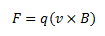Here, the magnetic force is directed towards the center of circular motion undergone by the object and acts as a centripetal force. Thus, if v and B are perpendicular to each other, the particle describes a circle. In other cases, when a component of velocity is present along the direction of the magnetic field B, then its magnitude remains unchanged throughout the motion, as no effect of a magnetic field is felt upon it. Also, the motion due to the perpendicular component of the velocity is circular in nature, as discussed above. The resulting motion due to the two components is a helical motion, as shown in the image below.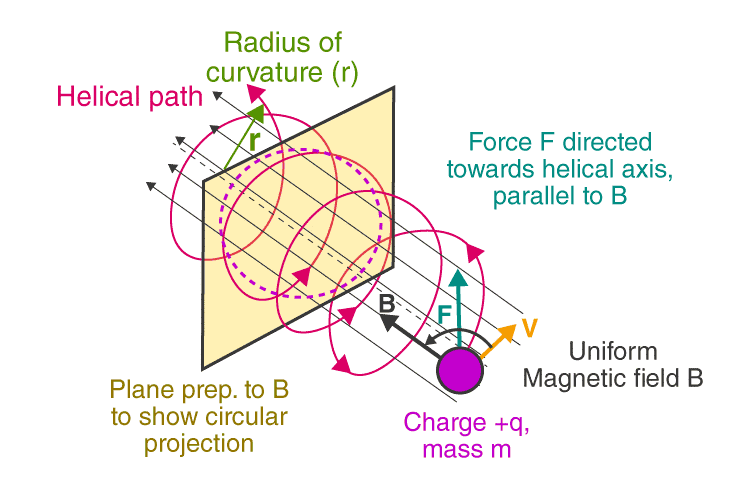As the radius of the circular path of the particle is r, the centripetal force acting perpendicular to it towards the center can be given as,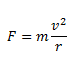Also, the magnetic force acts perpendicular to both the velocity and the magnetic field and the magnitude can be given as,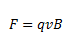Equating the two, we get,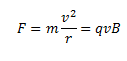Or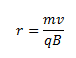Here, r gives the radius of the circle described by the particle. Also, if we write the angular frequency of the particle as ω, then we can write,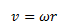So,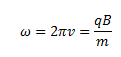Here, v is the frequency of rotation of the particle. The time for one revolution can be given as,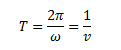The distance moved by the particle along the direction of the magnetic field in one rotation is given by its pitch.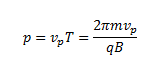Where vp is the velocity parallel to the magnetic field.

The document Motion in a Magnetic Field Notes | Study Physics Class 12 - NEET is a part of the NEET Course Physics Class 12.
All you need of NEET at this link: NEET

## Physics Class 12

157 videos|425 docs|213 tests
 Use Code STAYHOME200 and get INR 200 additional OFF

## Physics Class 12

157 videos|425 docs|213 tests

### How to Prepare for NEET

Read our guide to prepare for NEET which is created by Toppers & the best Teachers

Track your progress, build streaks, highlight & save important lessons and more!

,

,

,

,

,

,

,

,

,

,

,

,

,

,

,

,

,

,

,

,

,

;